﻿ Influence of Irradiation on the Diffusion’s Capacity of a Bifacial Solar Cell Containing Thin Film CIGSPublications are Open
Access in this journal
Article Versions
Export Article
• Normal Style
• MLA Style
• APA Style
• Chicago Style
Research Article
Open Access Peer-reviewed

### Influence of Irradiation on the Diffusion’s Capacity of a Bifacial Solar Cell Containing Thin Film CIGS

Salif CISSE , Alain EHEMBA, Ousmane DIAGNE, Mouhamadou Mamour SOCE, Moustapha DIENG
American Journal of Materials Science and Engineering. 2018, 6(1), 12-17. DOI: 10.12691/ajmse-6-1-3
Published online: April 28, 2018

### Abstract

The objective of this study is to present and explain theoretically the influence of irradiation energy on the diffusion capacity of a bifacial CuInGaSe2 solar cell under monochromatic illumination in dynamic frequency regime. Using the equation of continuity of the minority charge carriers in the base, the density of these carriers is determined. Then the expressions of the phototension are deduced. From these quantities the expressions of the capacitance are calculated and the representations of the curve of these parameters as well as the representation of the BODE diagram are interpreted. The obtained values are proposed with the effect of the irradiation energy.

### 1. Introduction

The functioning of the solar cells depends strongly on several external parameters, namely the effects of irradiation (related to the operating environment), influencing the energy conversion efficiencies of the photovoltaic solar cells. For reliable and viable storage and distribution of electricity, it is useful to characterize these various parameters 1. The irradiation energy that is the object of this study is an extremely important parameter and cannot be neglected in the behavior of solar cells. Hence the importance of studying the influence of irradiation energy on electrical parameters is to optimize the performance of photovoltaic systems since they are exposed to solar radiation. We propose first in this paper the study of the diffusion capacity of an n+/p/p+ bifacial CIGS photopile under monochromatic illumination, then we study the influence of irradiation energy in dynamic frequency regime and finally we carry out the study with one illumination by the front face.

### 2. Model of the Solar Cell

The study is based on a one-dimensional bifacial photovoltaic CIGS. Thus, a one-dimensional bifacial photopile is such that the illumination occurs perpendicularly at the level of the junction. We have considered an n+/p/p+ type photopile presented in the Figure 1.

In the framework of our study, we will make the following approximations:

- The transmitter's contribution to photocurrent will be neglected compared to that of the base station.

- The photopile is studied in a single dimension with the axis origin taken at the junction (x = 0) 2.

- The crystalline field will be neglected at the base of the photovoltaic cell and only the electric field at the junction will be taken into account 2.

• Figure 1. Bifacial photopile with CIGS type n+/p/p+

When the light source is illuminated, the evolution of excess minority carriers in the base is governed by the following equation of continuity in dynamic frequency regime: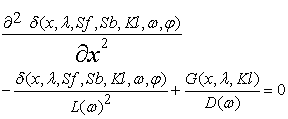(1)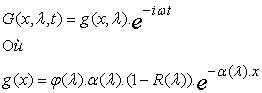(2)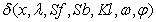, is the density of the electrons generated at the depth x in the base.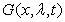is the generation rate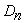is the diffusion coefficient of the minority carriers generated in the base and is related to the flux of irradiating particles as well as to the coefficient of damage by the relation (Francis Levy, 1995) 3.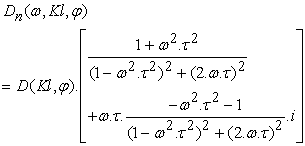(3)

Where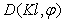is the diffusion coefficient related to the irradiation energy and the coefficient of damage and is given by the following relation: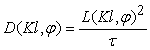(4)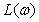Is the diffusion length of the excess minority carriers in the base of the photovoltaic cell. This diffusion length is related to the flux of irradiating particles and to the coefficient of damage by the following equation 7: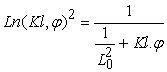(5)

L0 is the diffusion length before irradiation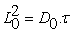(6)

is the lifetime of minority carriers of charge.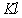is the coefficient of damage and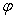, irradiation energy.

According to the different modes of illumination, the generation rate is given as following expressions:

• Front illumination 4.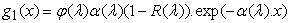(7)

• Rear illumination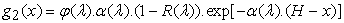(8)

• Simultaneous illumination of the two faces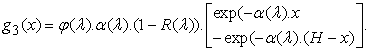(9)

Equation (1) is a second-degree differential equation with a second member.

The solution of this equation without a second member is: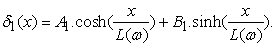(10)

As for the particular solution of this equation, it is of the form: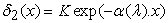(11)

Where K is a constant deduced from (1), (8) and (9):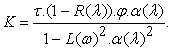(12)

The general solution, according to whether the photovoltaic unit is illuminated by the front face, by the rear face or simultaneously by the two faces, can be made in the form: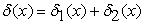, from where:

• Front illumination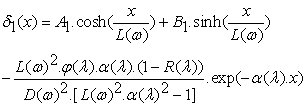(13)

• Rear illumination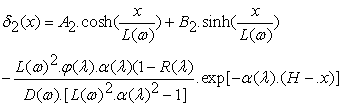(14)

• Simultaneous illumination of the two faces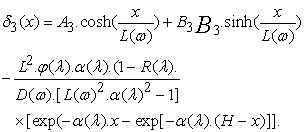(15)

The constants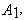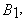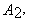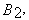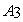and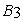are determined from the following boundary conditions 5:

√ At the junction (x = 0):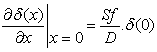(16)

Sf designates the recombination velocity of the carriers at the junction and translates the flow of the carriers which pass through the junction.

√ At the back (x = H):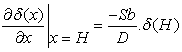(17)

Sb denotes the recombination velocity of the carriers at the rear face and reflects the manner in which the carriers are lost at the rear interface of the photovoltaic cell. So in this present work, we will work for an illumination at the front only.

2.1. Diffusion Capacity

In this section, we perform a modeling study of the capacitance as a function of the operating point of the photovoltaic cell.

The capacity of the photopile results from a variation of charge during the diffusion process within the photovoltaic cell. Its expression is given by 6: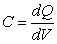(18)

The expression of the charge Q may take the form: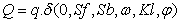Where q is the electrical charge. We have: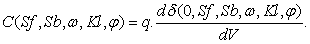(19)

The capacity can be expressed as a function of the rate of recombination at the junction which fixes the operating point and we have: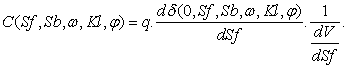(20)

Taking into account the expression of the density of the minority carriers written in (14) and that of the phototension written in the following form 6: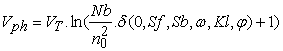(21)

Where VT is the thermal tension, Nb = 1016 (cm-3) the doping rate, n0 is the intrinsic density of the minority carriers in the base 7: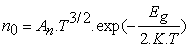(22)

With An a constant. An = 30.87x1016 cm-3 K-3 /2, Eg = 1,04x1, 6.10-19 J, the gap.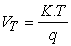Is the thermal voltage.

We deduce the expression of capacity in the following form: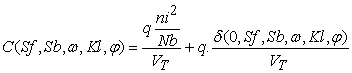(23)

We pose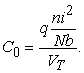C0 is the intrinsic capacity, it depends on the nature of the material (substrate) through (ni), the doping of the material through Nb and the temperature through VT.

The second term of equation (23) depends on the temperature through VT, the doping of the material, and its nature through the diffusion coefficient D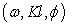and the diffusion length L, Of the operating point (Sf), the quality of the back interface (Sb), and the dimension of the photovoltaic cell (H = 3 μm).

Thus we can already admit that the diffusion capacity can be easily influenced by irradiation, according to this analysis of its expression.

2.2. Results and Discussions
2.2.1. Effect of Irradiation Energy on the Capacity of the Solar Cell

We illustrate in Figure 2 below the profile of the diffusion capacity as a function of the rate of recombination at the junction for various values of irradiation energy.

• Figure 2. Diffusion capacity profile as a function of the recombination rate at the junction for different irradiation energy values;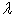=740nm, Sb=3x103cm.s-1, =5x105 Hz, Kl=5 cm2.s

The diffusion capacity decreases when the irradiation energy becomes more and more important, which means that the degradations are more predictable when there is an accumulation of a large load. There is also a decrease in capacity with the operating point. Indeed, for small values of the recombination speed at the junction (Sf), corresponding to the open circuit, the capacitance reaches a maximum value. Then, it decreases until it reaches a zero value for high recombination velocities at the junction, corresponding to the short circuit.

In open circuit (low values of Sf), the charge carriers generated do not cross the junction. They are stored on either side of this junction. The junction can thus be assimilated to a capacitance plane capacitor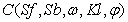.

In short-circuit (large values of Sf), the carriers generated in the photovoltaic cell cross the junction and are collected by the grids. In the latter case, there is no storage of charge, hence a low capacity.

From the relation (21), we note that the capacitance is a function of the voltage across the photovoltaic cell. We have therefore studied the evolution of the capacitance as a function of the voltage of the solar cell. The Figure 3 is a representation of the evolution of the capacitance as a function of the voltage of the solar cell.

The curves of the Figure 3 show the appearance of the capacitance as a function of the phototension of the photovoltaic cell when it is illuminated by the front face for various irradiation energies.

• Figure 3. The profile of the diffusion capacity as a function of the voltage for different irradiation energy values and for different values of Sf;= 740 nm, Sb = 3x10-3 cm.s-1,= 5x105 Hz, Kl=5 cm2.s-1

It is noted that, according to the curves, the diffusion capacity increases with the voltage. Indeed, when the voltage of the photovoltaic cell is maximum: open circuit operating point (respectively, minimum: short circuit operating point) corresponds to the open-circuit capacitance of the photovoltaic cell (respectively corresponds to the capacitance of diffusion of the photovoltaic cell in short-circuit).

On the other hand, we note a decrease in the capacity with the irradiation energy, which is estimated, because the irradiation energy affects the value of the photovoltaic capacity, which is in good agreement with the results of the (Figure 2).

We represent in Figure 4 the logarithm of the diffusion capacity as a function of the voltage of the photovoltaic illuminated by its front face.

• Figure 4. Logarithm of the diffusion capacity as a function of the voltage;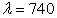nm, Sb = 3x103 cm.s-1, Kl= 5 cm2.s-1,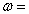x105 Hz

We note that the logarithm of the capacitance is a linear function of the voltage of the photovoltaic cell, whose slope is strictly positive. This justifies that the capacity increases with the voltage, as observed previously in the Figure 3.

It should also be noted that the capacity always decreases with the irradiation energy, but this time it varies slightly (Figure 4).

This study makes it possible to determine at V = 0 Volt, the diffusion capacity of the photo voltage in short-circuit and at V = Voc the capacity of diffusion in open circuit.

2.2.2. Effect of Irradiation on Capacity Efficiency

By assimilating the space charge region with a planar capacitor, the expression of the efficiency of the solar cell capacity is given by the following equation 8: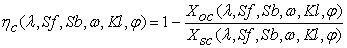(24)

Where Xsc is the abscissa of the density of the short-circuit current and Xoc, that of the open circuit.

In addition, the capacitance of the short-circuit and open-circuit photovoltaic cell is written 9: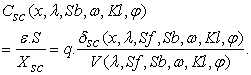(25)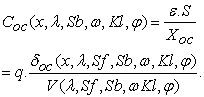(26)

S is the area of the cell; S = 1 cm2,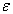is the permittivity;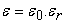, where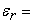13.6 is the permittivity of the CIGS and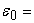8.85x10-14 F.cm-1 is the permittivity of the vacuum.

We have assumed that the junction is under constant potential. Thus, by extracting the expression of and from equations (25) and (26) and referring to equation (24), we obtain a new expression of the efficiency of the diffusion capacity given by:(27)

Where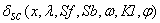and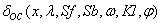represent respectively the relative density of the excess minority carriers in short-circuit and open circuit conditions.

The appearance of the relative density of excess minority carriers in short-circuit and open-circuit condition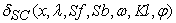and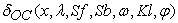is obtained from Figure 5.

• Figure 5. Profile of the relative density of minority charge carriers in excess in the base as a function of the depth x of the base for different points of operation;=740 nm, Sb = 3x103 cm.s-1,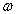= 5x105 Hz, Kl= 5 cm2.s-1,= 25 MeV

We notice from this figure that there exists a point X0 in the depth of the base where the density of the excess carriers has a zero gradient. This point moves in the depth of the base, when the rate of recombination at the junction Sf increases. Thus, regions where charge carrier density has a positive gradient can be likened to an extension of the space charge region (SCR) of capacitance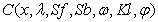, to the volume of the database.

To determine this enlargement of the SCR, we solve the following equation: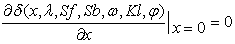(28)

The values of Xcc and Xco are obtained by the resolution of equation (28) allowing us to deduce the different values of the capacitance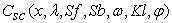and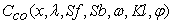corresponding to equations (25) and (26).

We show in the Figure 6 the profile of the efficiency of the capacity of the solar cell as a function of the depth of the base, for different values of irradiation energy and for a given coefficient of damage.

• Figure 6. Variation of the efficiency of the diffusion capacity according to the thickness of SCR, for different values of irradiation energy,=740 nm, Sb = 4x104 cm.s-1,=5x105 MeV, Kl =5 cm2.s-1

The efficiency of the capacity decreases rapidly with the thickness of the space charge area. Indeed, the enlargement of the SCR leads to a reduction in capacity, whereas the narrowing of the SCR causes an increase in capacity, which explains this maximum capacity for a small thickness of the SCR and a decrease in capacity for a large thickness of the SCR.

On the other hand, the efficiency of the capacitance decreases with the irradiation energy from a thickness Xs of the space charge region. Indeed, we have shown in Figure 2 that the irradiation energy causes a decrease in capacity, hence a decrease in its efficiency.

2.2.3. BODE Diagram of the Modulus of Diffusion Capacity in Short Circuit Condition

The BODE diagram of the diffusion capacity consists of plotting the capacity modulus as a function of the logarithm of the excitation frequency.

In the following Figure 7, the BODE diagram of the diffusion capacity for different irradiation energy values is shown.

For the angular frequencies in the interval 0 <<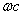we are in quasi-static mode, i.e.: the module of the capacitance is independent of the frequency. Above that of>, the module of capacity decreases with frequency. Thus, the cut-off frequency) can be determined from the intersection of the tangents of the curve, as shown in the Figure 8.

Knowing the values of the cut-off pulsation ωc and the capacitance, the following relation allows us to determine the parallel resistance (Rp) 9: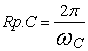(29)
• Figure 7. Variation of the Module of the capacitance according to the logarithm of the frequency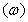, for different values of irradiation energies;= 740 nm, Sf = 5x105 cm.s-1, Sb = 3x103 cm.s-1, Kl=5cm2.s-1
• Figure 8. Variation of the modulus of capacitance according to the logarithm of the frequency (),= 0.1 MeV,= 740 nm, Sf = 5x105 cm.s-1, Sb = 3x103 cm.s-1, Kl= 5 cm2.s-1

Thus, the Table1 gives the values of the cut-off frequency, the parallel resistance and the scattering capacity according to the irradiation energy.

From this table, we notice a decrease in the cut-off pulsation, the scattering capacity as well as the parallel resistance with the increase of the radiation energy in frequency modulation. This further confirms the degrading effect of the irradiation energy with respect to the electrical parameters of the photovoltaic cell.

### 4. Conclusion

A theoretical study of a bifacial solar cell with horizontal junction under monochromatic illumination in frequency modulation was presented in this work. However, the study allowed us to show that the increase in irradiation energy leads to a decrease in the diffusion capacity, as well as the efficiency of the latter. The results obtained from the BODE diagram confirmed that the diffusion capacity is sensitive to the effect of the irradiation energy which is a very important parameter in the behavior of the solar cells. Indeed, the study of the dependence of the diffusion capacity of thin-film solar cells based on CuInGaSe2 is therefore very important.

### References

  A. Diao, M. Wade, M. Zoungrana, M. Sarr, M. Ndiaye, N. Thiam, A. Thiam, A. Dieng, M. Ngom, A. S. Maiga, G. SISSOKO «Equivalent electrical models in the dynamic frequency regime of the impedance of a bifacial photopile under the effect of a constant magnetic field for polychromatic illumination by the rear face», Sciences Journal Vol. 11, N°1 (2011) pp: 20-26. In article  R. K. Ahrenkiel, D. J. Dunlavy, H. C. Hamaker, R. T. Green, C. R. Lewis, R. E. Hayes, H. Fardi « Time of-flight studies of minority-carrier diffusion in AlxGa1-xAs homojunctions » J. Appl. Phys. 49(12) (1986). In article View Article  El Hadji Ndiaye, Gokhan Sahin, Moustapha Dieng, Amary Thiam, Hawa Ly Diallo, Mor Ndiaye, Grégoire Sissoko, .« Study of the Intrinsic Recombination Velocity at the Junction of Silicon Solar under Frequency Modulation and Irradiation », Journal of Applied Mathematics and Physics (3), pp : 1522-1535 (2015). In article  M.M. Dione, H. Ly Diallo, M. Wade, I. Ly, M. Thiame, F. Toure, A. Gueye Camara, N. Dieme, Z. Nouhou Bako, S. Mbodji, F. I Barro, G. Sissoko «Determination of the shunt and series resistances of a vertical multijunction solar cell under constant multispectral light» 26th European Photovoltaic Solar Energy Conference and Exhibition pp: 250-254, (2011). In article  M. A. Ould EL Moujtaba, M. Ndiaye, A. Diao, M. Thiam, I.F. Barro and G. Sissoko « Theorical Study of Influence of Irradiation on a Silicon Solar Cell Under Multispectral Illumination », Researche Journal of Applied Sciences Engineering and Technology, Vol. 23, Issue 4, pp.5068-5073, (2012). In article  N fally Dieme, Martial Zoungrana, S. Mbodji, H. Ly Diallo, Mor Ndiaye, F. I. Barro and Grégoire Sissoko « Influence of Temperature on the Electrical Parameters of a Vertical Parallel Junction Silicon Solar Cell under Polychromatic Illumination in Steady State », Research Journal of Applied Sciences, Engineering and Technology 7(12): pp: 2559-2562, (2014). In article View Article  Ibrahima Diatta, Issaga Diagne, Cheikh Sarr, Khady Faye, Mor Ndiaye, Grégoire Sissoko, « Silicon Solar Cell Capacitance : Influence of Both temperature and wavelength », IPASJI International Journal of Computer Science (IIJCS) Vol.3, Issue 12 pp : 1-8, (2015). In article  Mouhamadou Moustapha DEME, Songdé SARR, Raguilnaba SAM, Séga GUEYE, Mamadou Lamine SAMB, Fabé Idrissa BARRO, Grégoire SISSOKO, « Influence of grain size, recombination rate at grain boundaries and angle of incidence of illumination on the widening of the space charge area of a monofacial photovoltaic », Sciences Journal Vol.9, N°2 (2009). pp: 17-27. In article  M. NDIAYE, Z. NOUHOU BAKO, I. ZEBRO, A. DIENG, F. I. BARRO, G. SISSOKO, «Determination of the electrical parameters of a photovoltaic cell under monochromatic illumination in frequency modulation, using the Bode and Nyquist diagrams », Sciences Journal Vol.8, N°3 (2008) pp: 59-68. In article

Published with license by Science and Education Publishing, Copyright © 2018 Salif CISSE, Alain EHEMBA, Ousmane DIAGNE, Mouhamadou Mamour SOCE and Moustapha DIENGThis work is licensed under a Creative Commons Attribution 4.0 International License. To view a copy of this license, visit http://creativecommons.org/licenses/by/4.0/

• Figure 5. Profile of the relative density of minority charge carriers in excess in the base as a function of the depth x of the base for different points of operation;=740 nm, Sb = 3x103 cm.s-1,= 5x105 Hz, Kl= 5 cm2.s-1,= 25 MeV